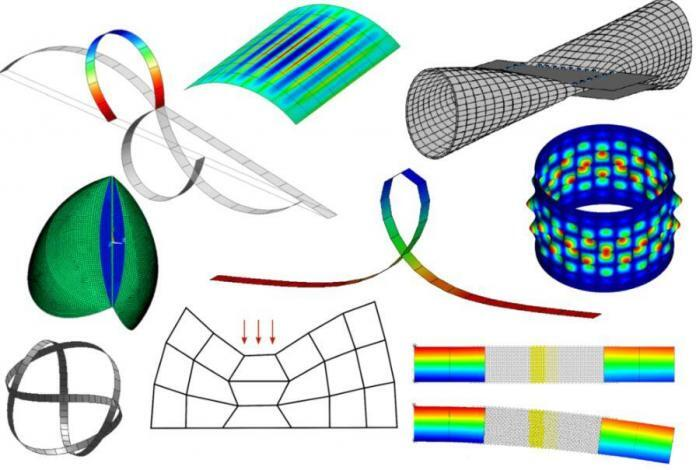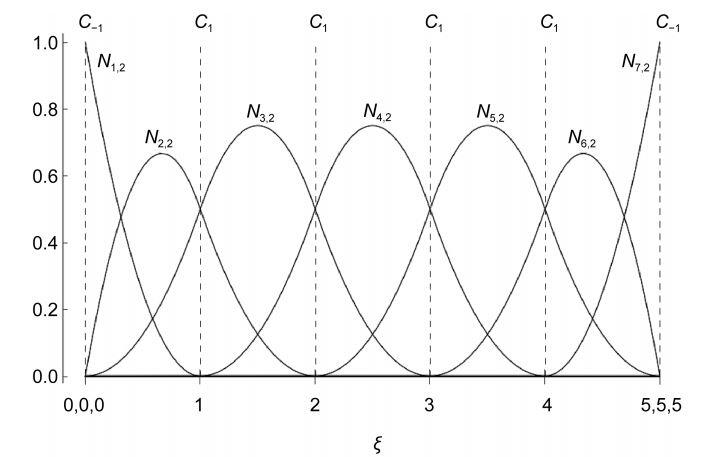# Modeling of Soils Using Isogeometric AnalysisResearchers from the Department of Construction Sciences, Lund University, Sweden have presented isogeometric analysis as an alternative to finite element analysis for modelling of soil plasticity. In a study published in the year 2017 in Geomaterials Journal, the researchers were able to show that isogeometric analysis showed good agreement with finite element method for drained soils in two- and three-dimensions. The research, therefore, suggested that isogeometric analysis is a good alternative to conventional finite element analysis for simulations of soil behavior.

Isogeometric analysis is a numerical method that uses non-uniform rational B-splines (NURBS) as basis functions instead of the Lagrangian polynomials often used in the finite element method. These functions have a higher-order of continuity and therefore makes it possible to represent complex geometries exactly. The basic idea behind isogeometric analysis is to use splines (NURBS) as basis functions for computational analysis by applying them directly. This allows the same basis function to be used for discretzation and for analysis.Representation of quadratic B-spline basis function with knot vector Ξ = {0, 0, 0, 1, 2, 3, 4, 5, 5, 5} (Spetz et al, 2017)

Since being introduced by Thomas J.R. Hughes at the University of Austin, Texas in the year 2005, isogeometric analysis has found numerous applications in engineering such as analysis of thin plates and shells, soil-structure interaction, fluid-structure interaction, flow through porous media, etc. However, finite element analysis has been used extensively for constitutive modelling of soils for the design of foundations, retaining walls, slope stability problems etc.

For the purpose of the research, Drucker-Prager criterion and the theory of plasticity was used by the researchers to evaluate the influence of the NURBS-basis functions on the plastic strains for granular materials against the conventional finite element analysis approach.

To compare the results of the findings, a 2D model of a strip footing on sandy silt was analyzed. The problem was solved for plane strain conditions using quadratic NURBS based IGA and conventional FEA with 5 different meshes. In order to compare the two methods, the element meshes were constructed using quadratic isoparametric elements for both IGA and conventional FEA.